## Introduction

This document is intended to help users of the mosaic package migrate their lattice package graphics to ggformula. The mosaic package provides a simplified and systematic introduction to the core functionality related to descriptive statistics, visualization, modeling, and simulation-based inference required in first and second courses in statistics.

Originally, the mosaic package used lattice graphics but now support is also available for the improved ggformula system. Going forward, ggformula will be the preferred graphics package for Project MOSAIC.

## Histograms

### Histograms (ggformula)

library(mosaic)   # also loads ggformula
gf_histogram(~ age, data = HELPrct)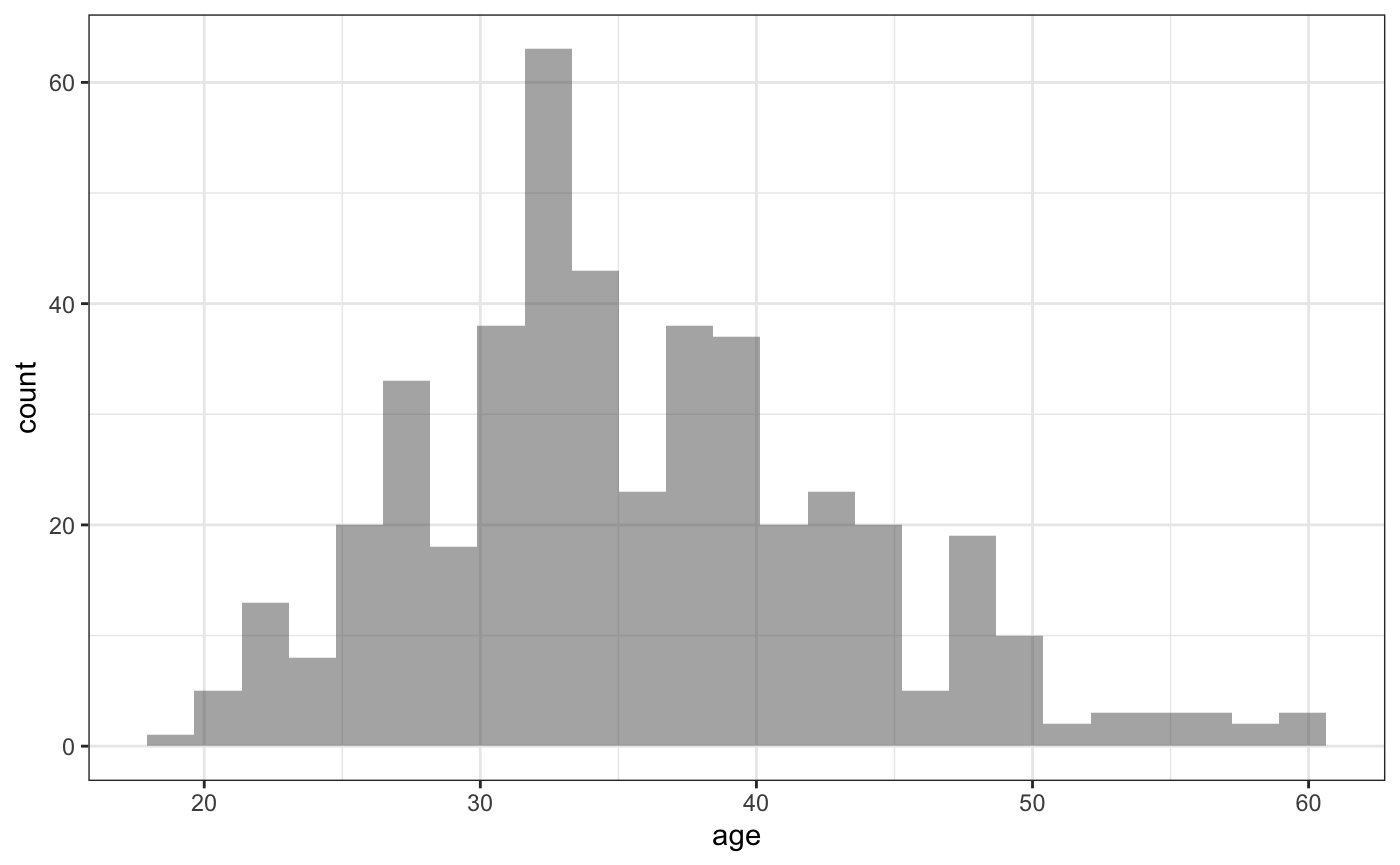### Histogram options (ggformula)

gf_histogram(~ age, data = HELPrct,
binwidth = 5)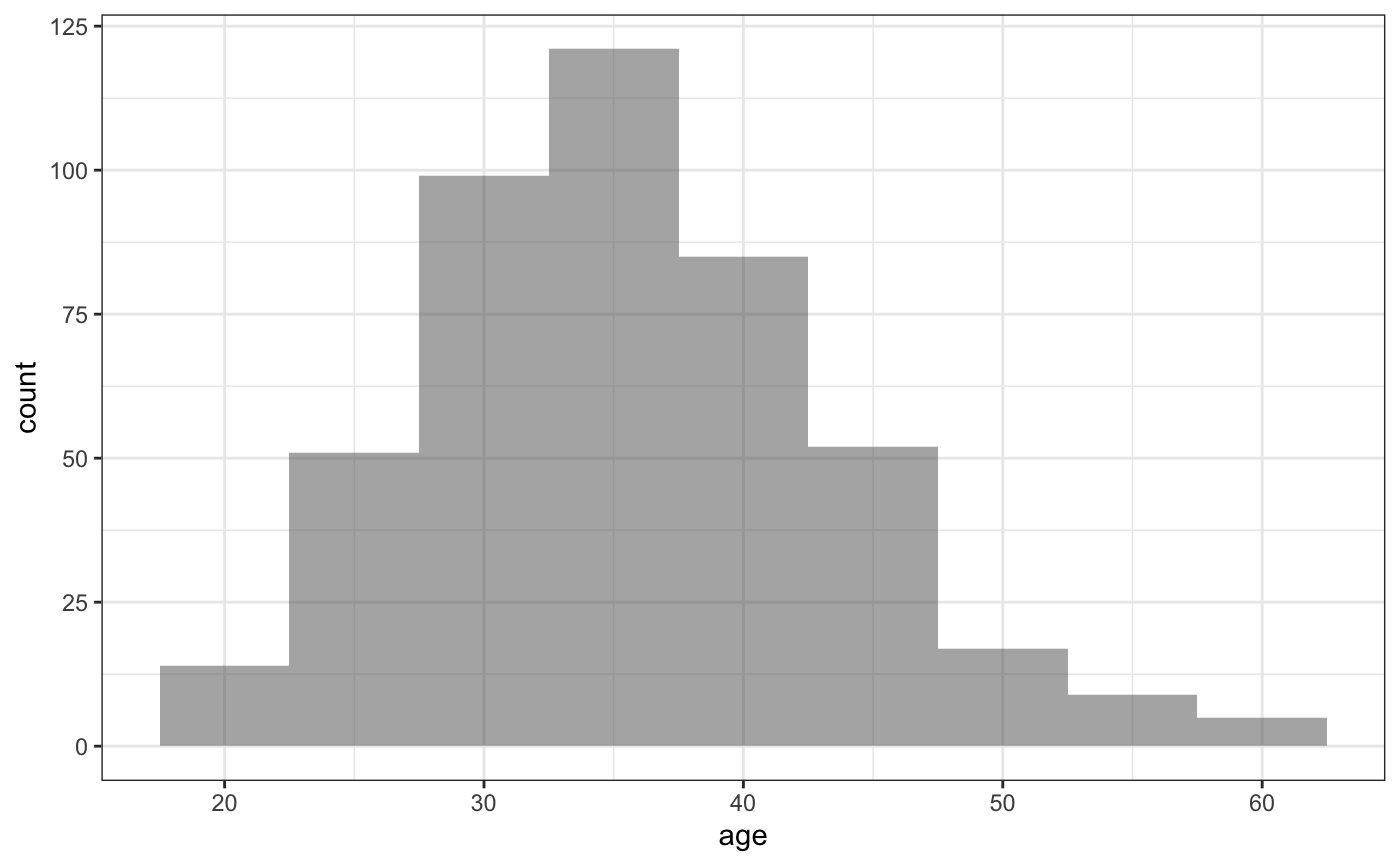### Histograms (lattice)

library(mosaic)     # also loads lattice
histogram(~ age, data = HELPrct)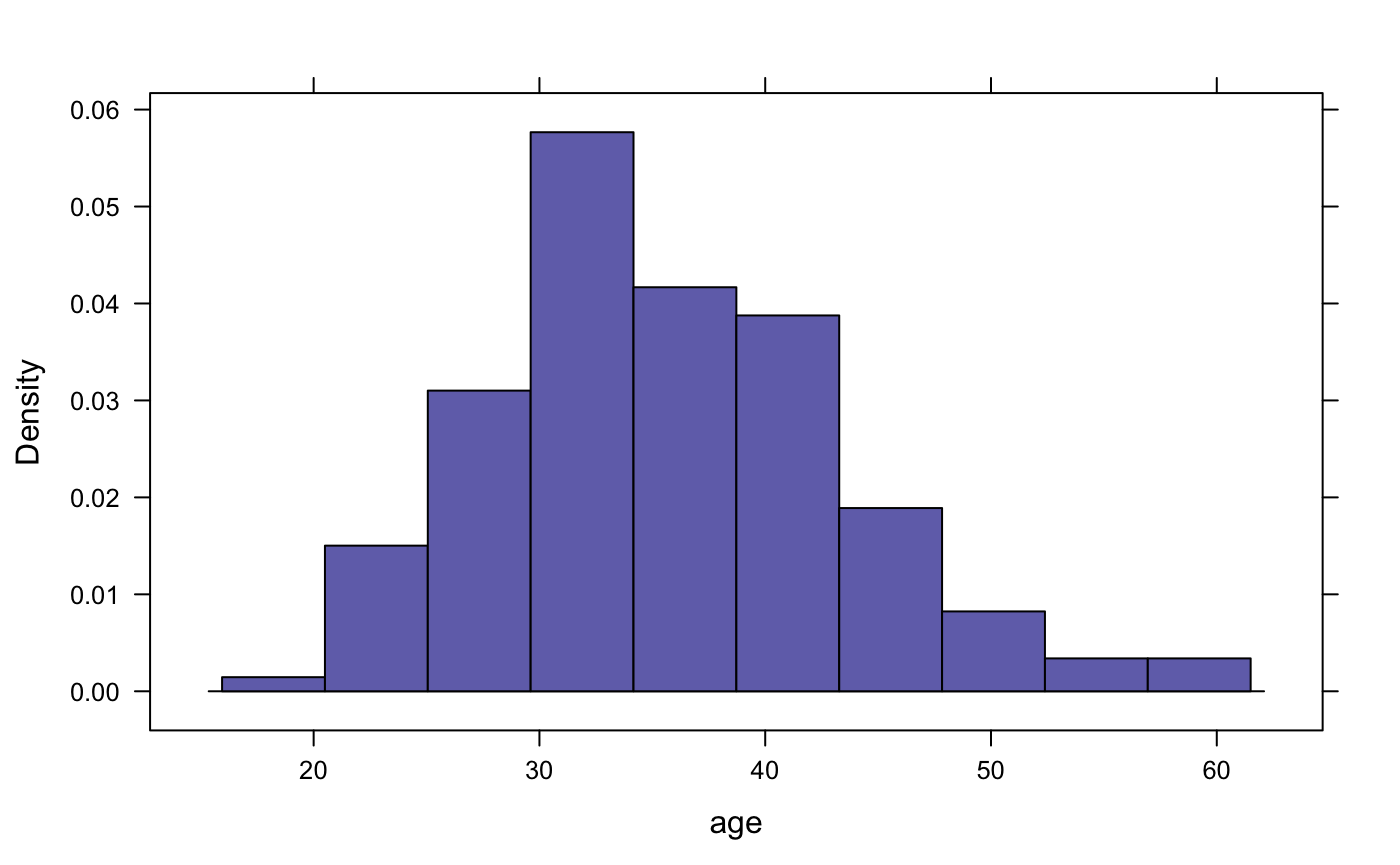### Histogram options (lattice)

histogram(~ age, width = 5, data = HELPrct)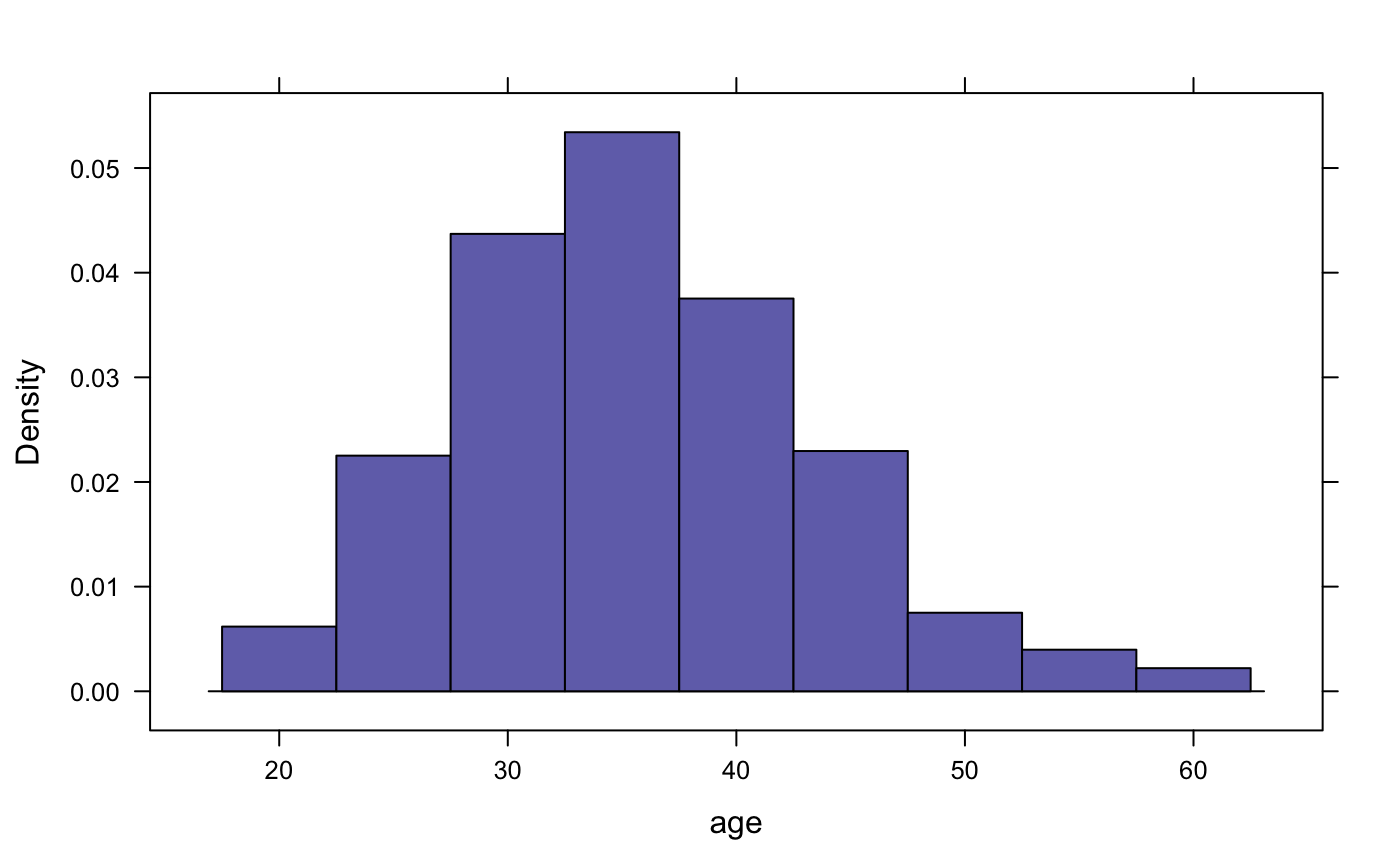## Density Plots

### Density plots (ggformula)

gf_dens(~ age, data = HELPrct)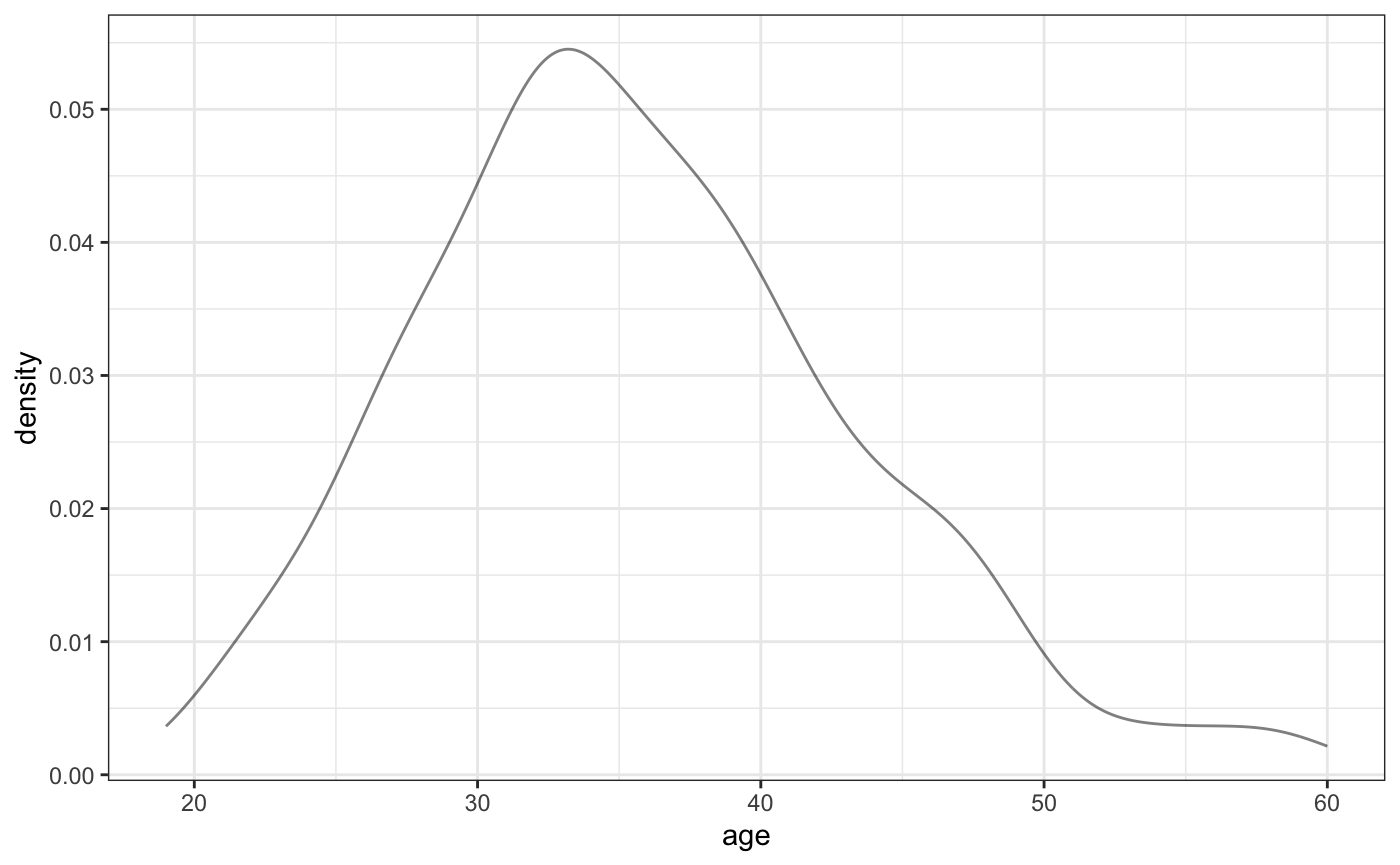### Overlaid density plots (ggformula)

gf_dens(~ age, data = HELPrct,
color = ~ sex)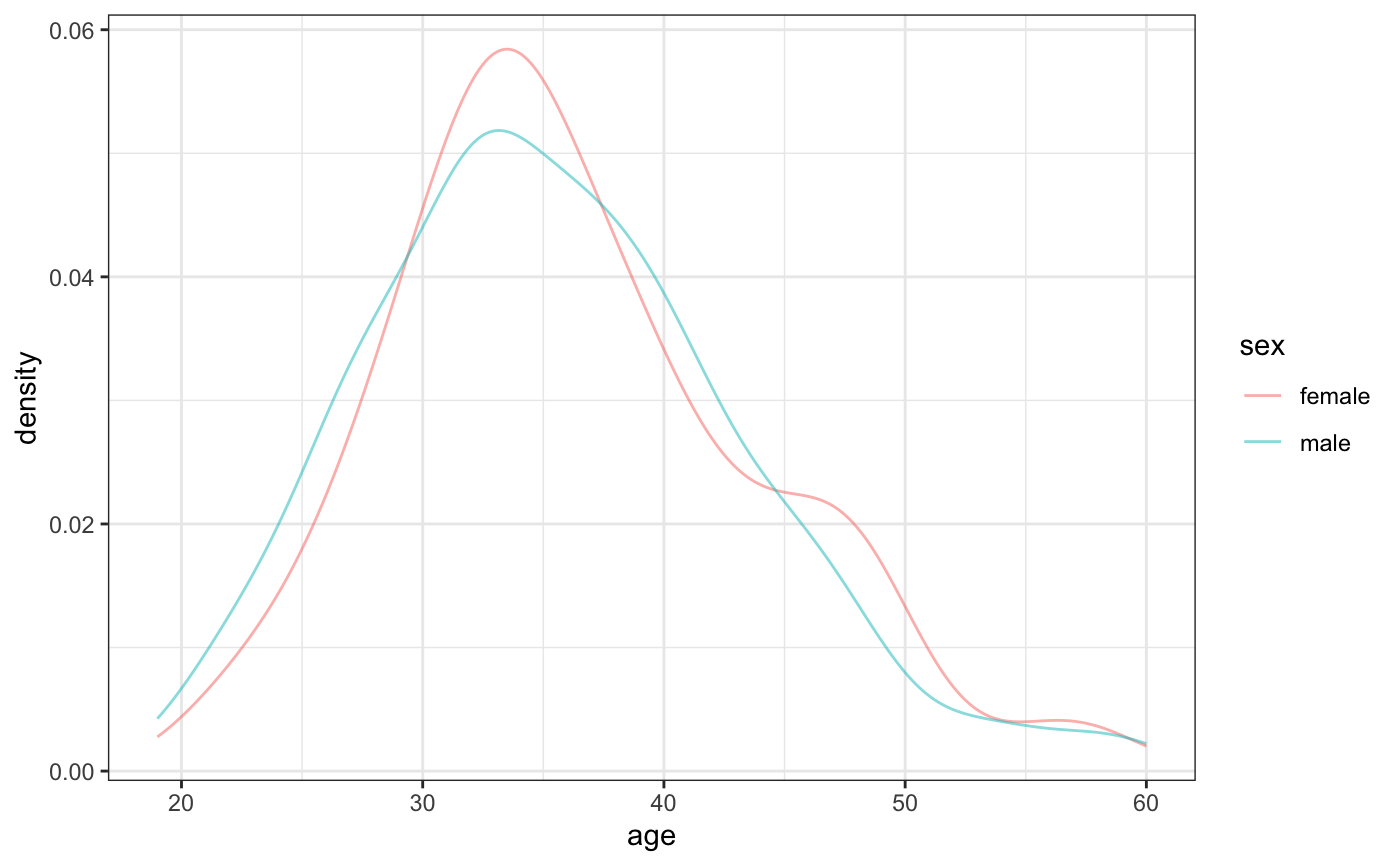### Density over histograms (ggformula)

We can use stacked layers to add a density curve based on a maximum likelihood fit or a kernel density estimate (see also gf_dist())

gf_dhistogram( ~ age, data = HELPrct,
alpha = 0.5) %>%
gf_fitdistr(color = ~"MLE", dist = "dnorm") %>%
gf_dens(color = ~"KDE")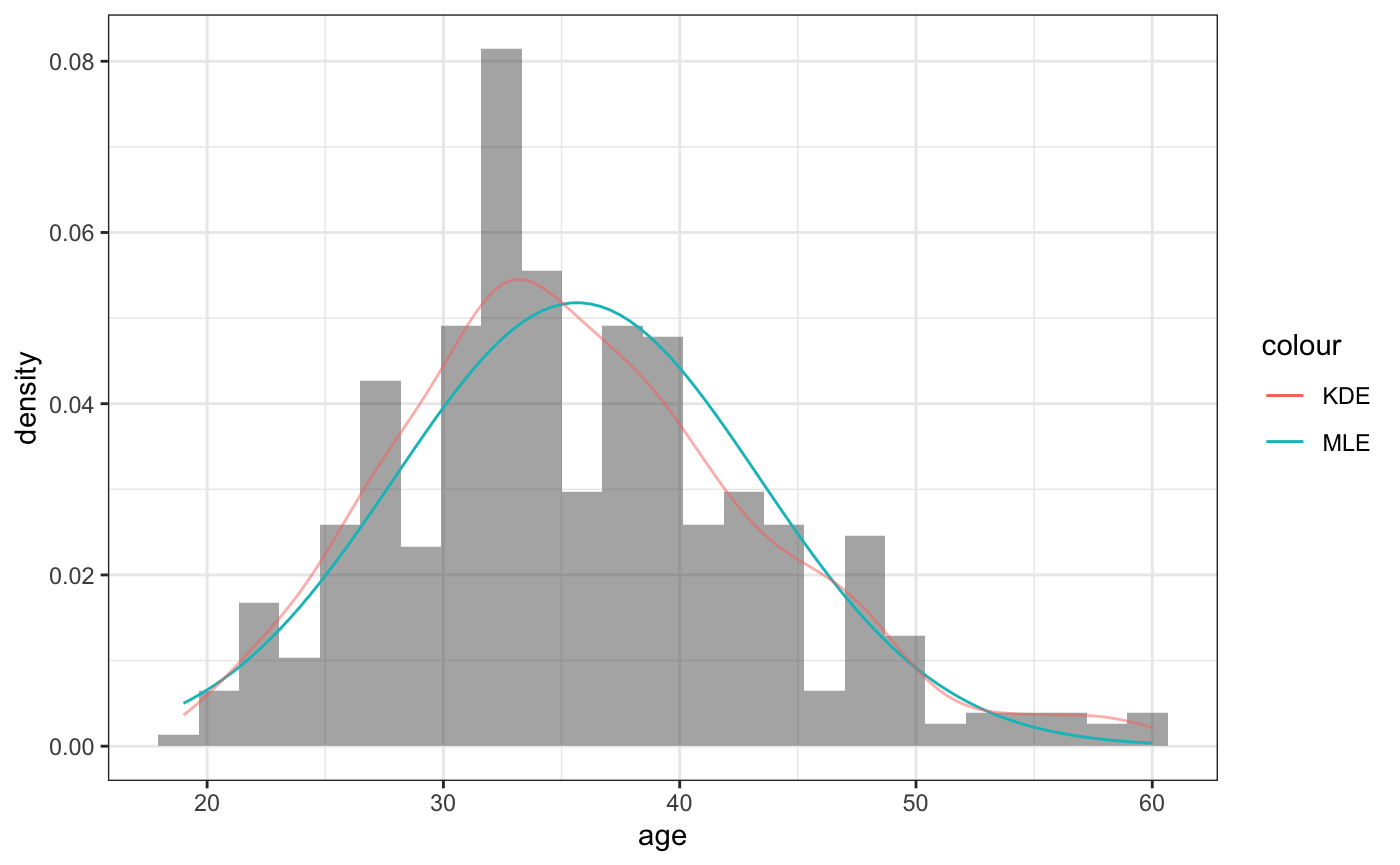### Density plots (lattice)

densityplot(~ age, data = HELPrct)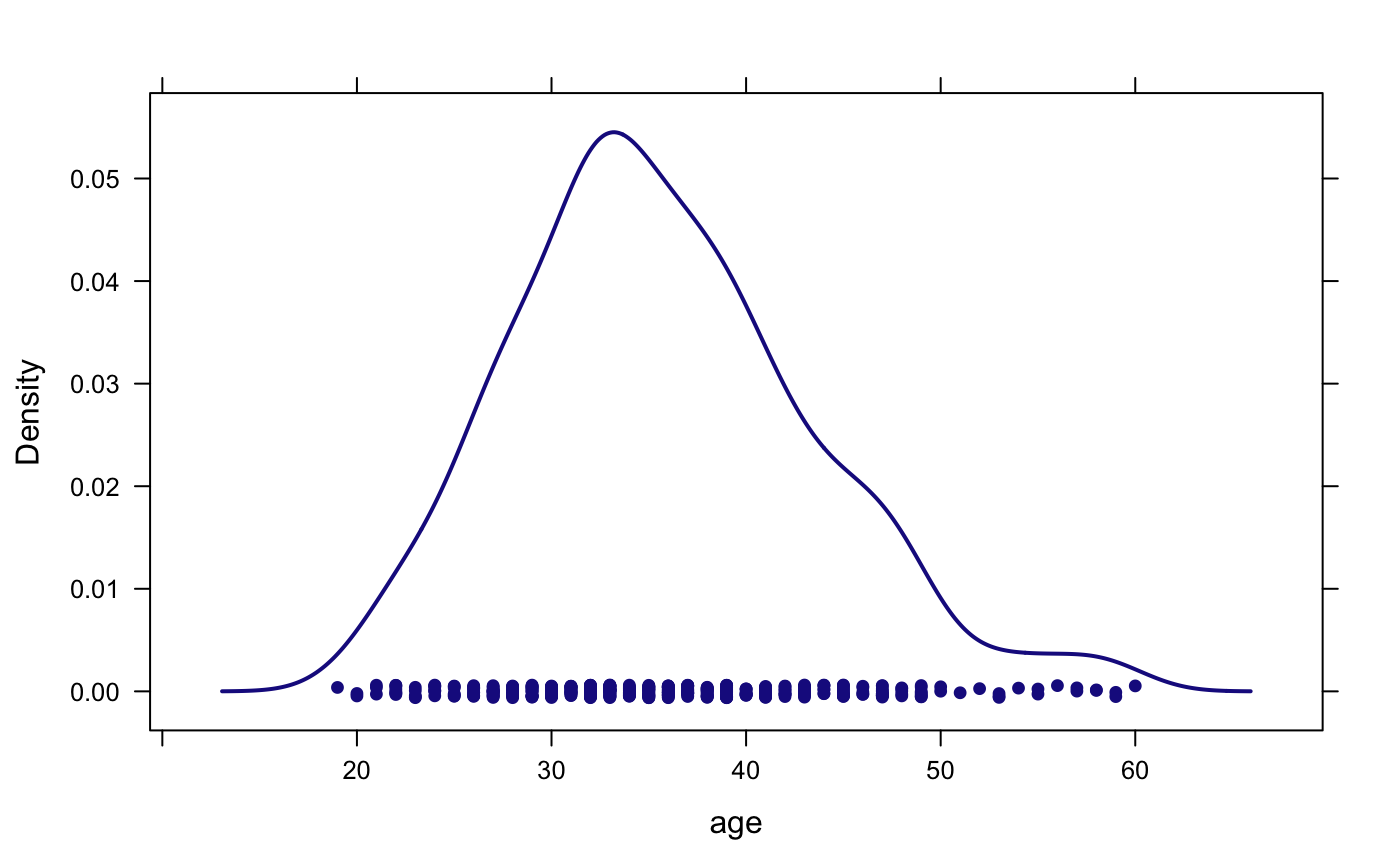### Overlaid density plots (lattice)

densityplot(~ age, data = HELPrct,
groups = sex,  auto.key = TRUE)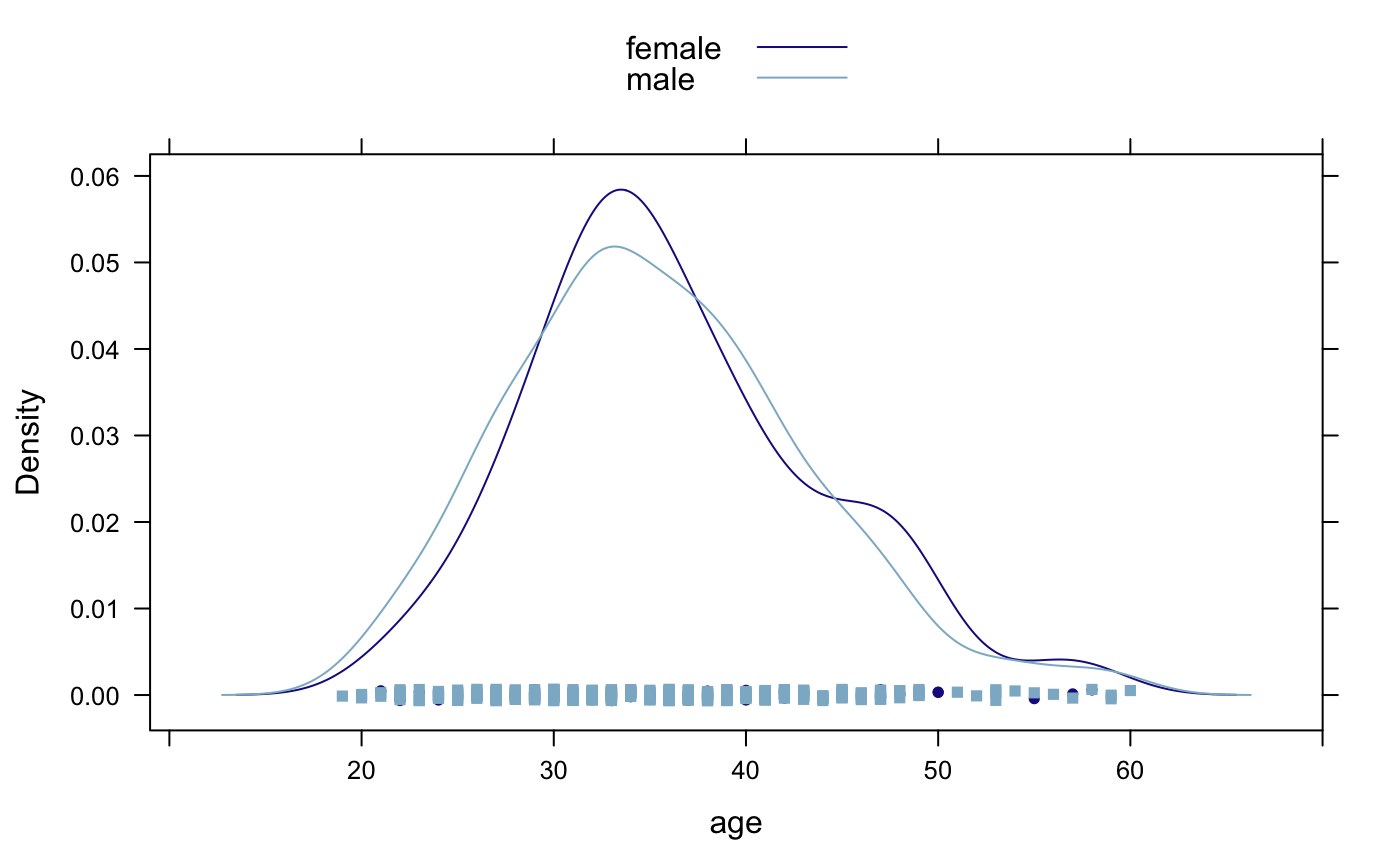### Density over histograms (lattice)

mosaic makes it easy to add a fitted distribution to a histogram.

histogram(~ age, data = HELPrct,
fit = "normal", dcol = "red")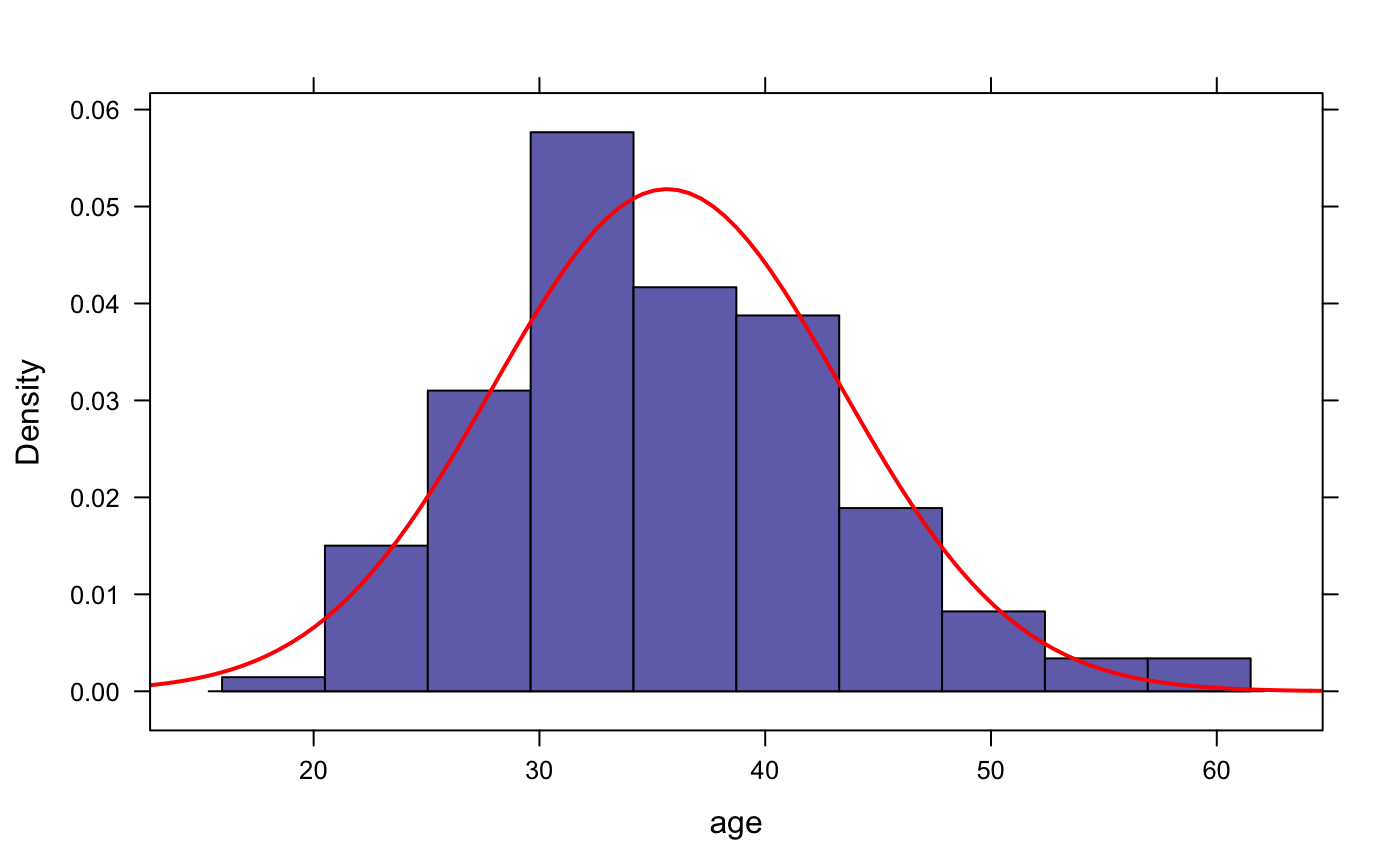## Side by side boxplots

### Side by side boxplots (ggformula)

gf_boxplot(age ~ sex, data = HELPrct)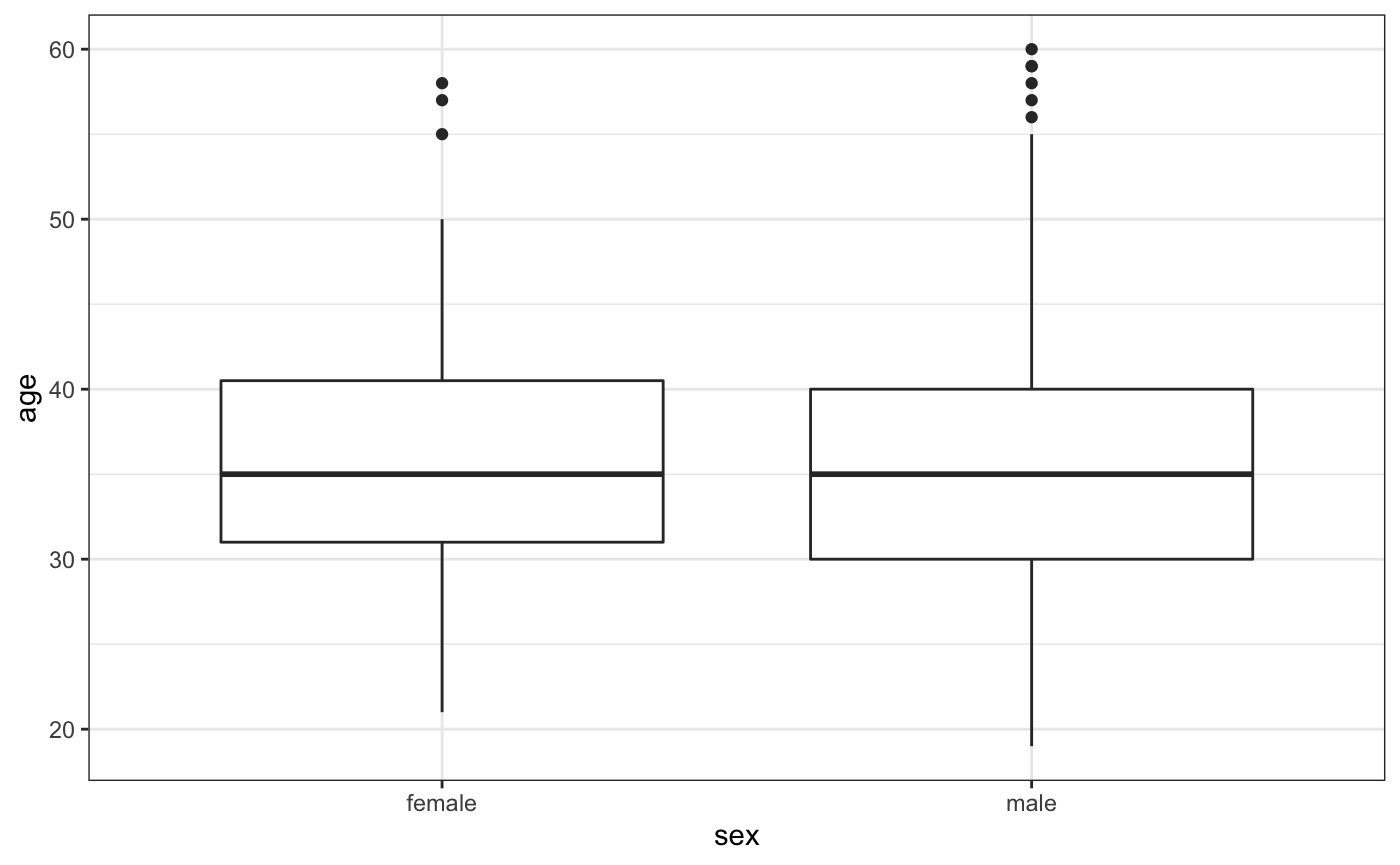### Faceted side by side boxplots (ggformula)

gf_boxplot(age ~ sex | homeless,
data = HELPrct)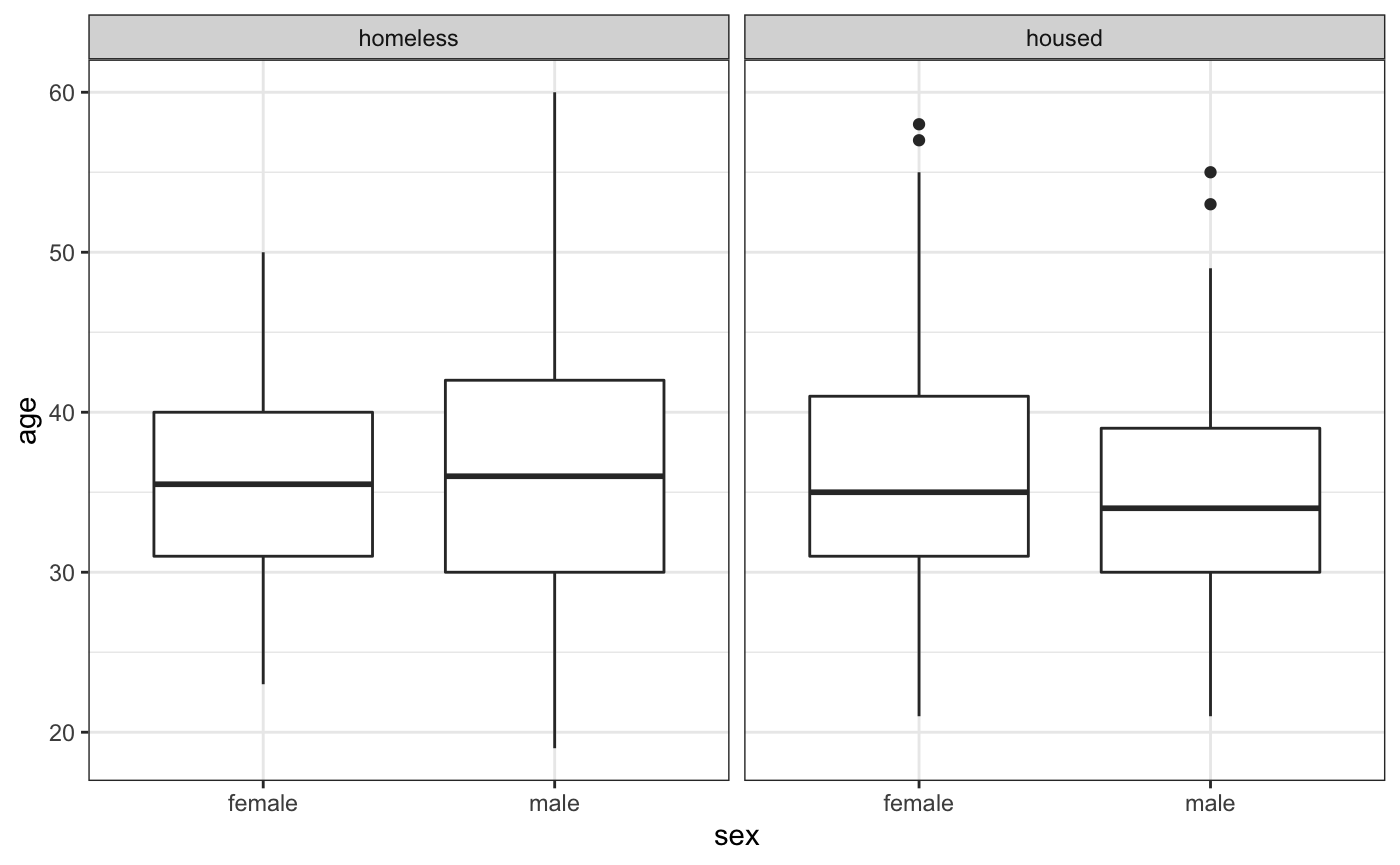### Horizontal boxplots (ggformula)

gf_boxploth(sex ~ age, data = HELPrct)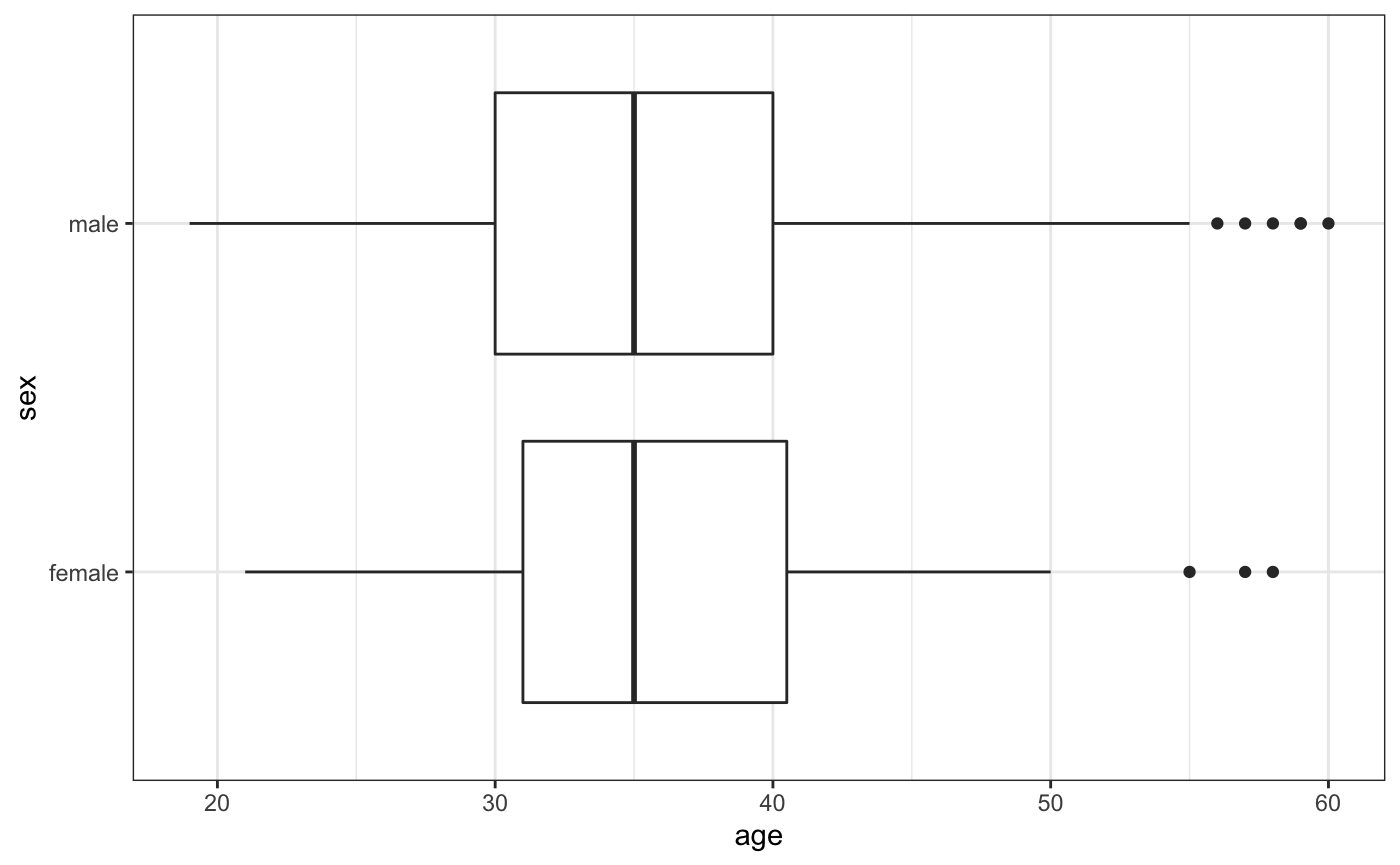### Side by side boxplots (lattice)

bwplot(age ~ sex, data = HELPrct)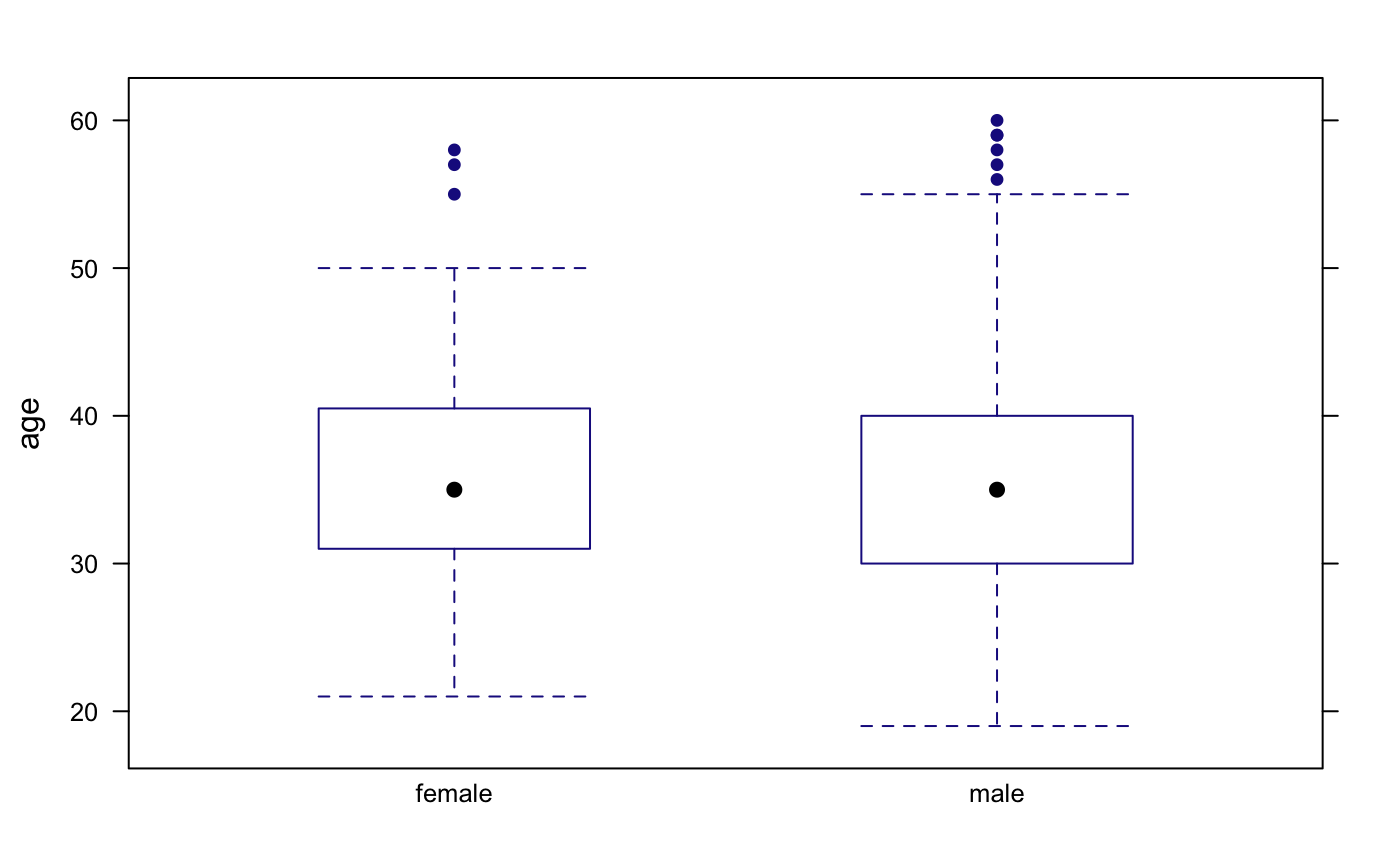### Faceted side by side boxplots (lattice)

bwplot(age ~ sex | homeless,
data = HELPrct)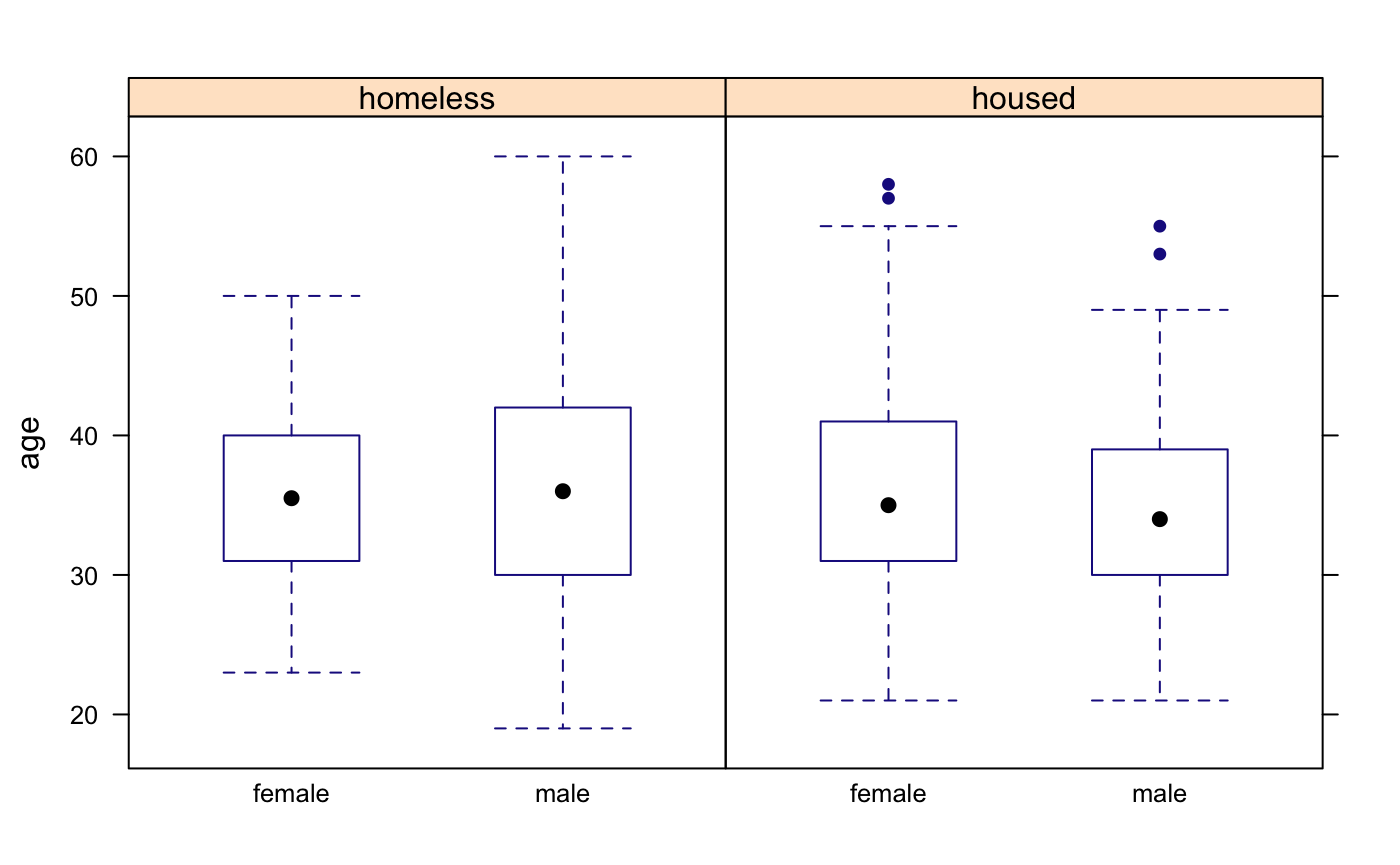### Horizontal boxplots (lattice)

bwplot(sex ~ age, data = HELPrct)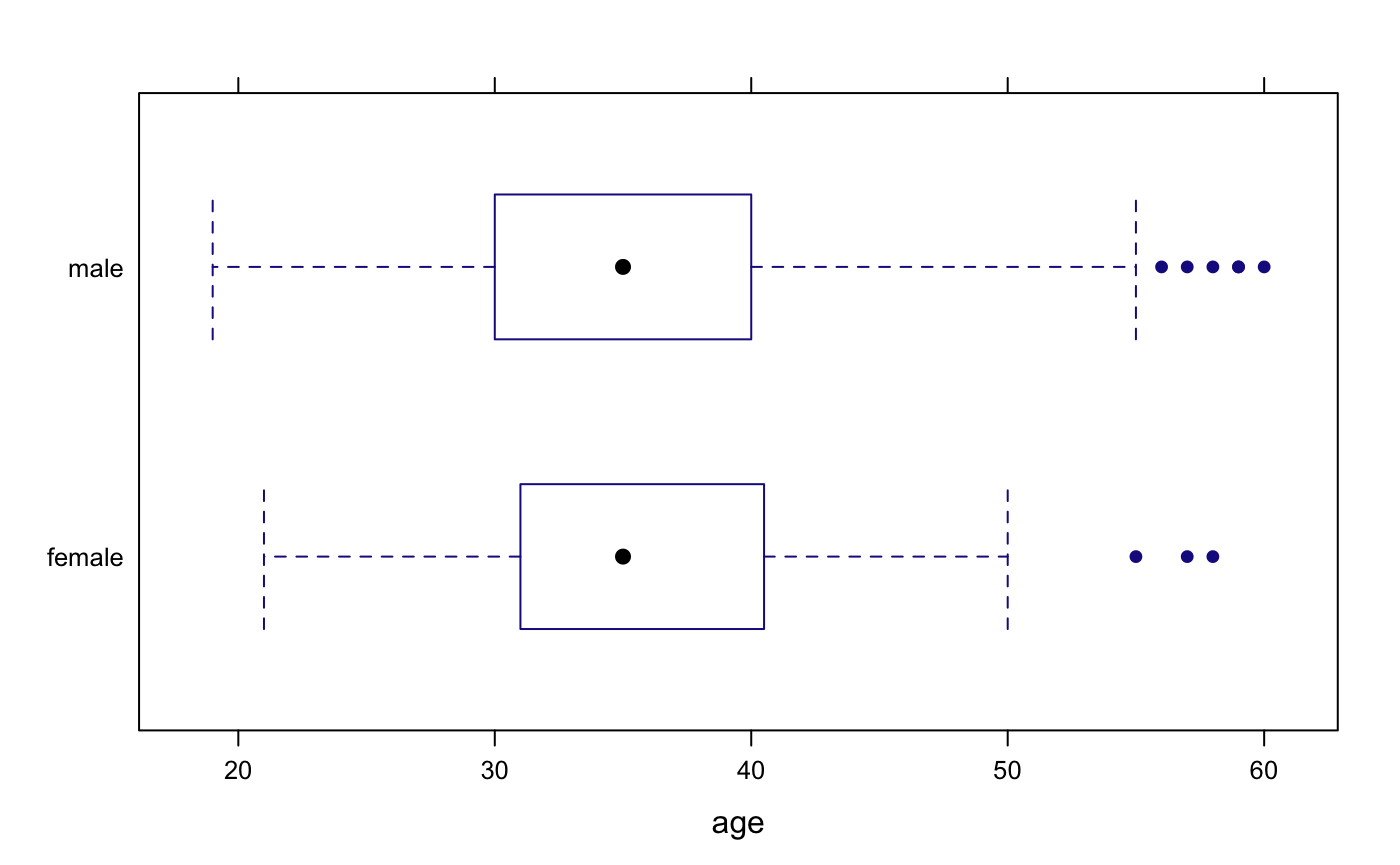## Scatterplots

### Basic scatterplot (ggformula)

gf_point(cesd ~ age, data = HELPrct)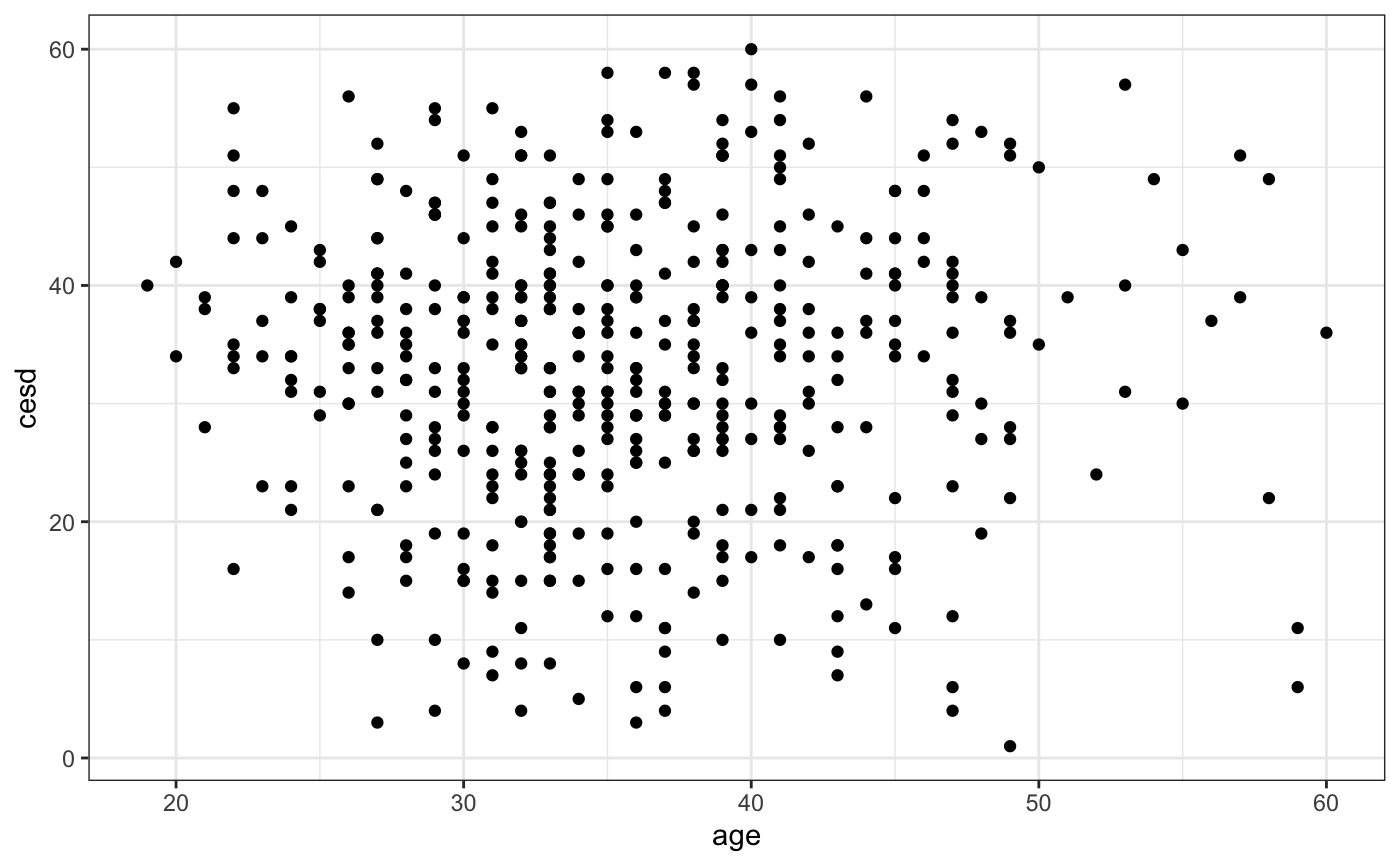### Overlaid scatterplot with linear fit (ggformula)

gf_point(cesd ~ age, data = HELPrct,
color = ~ sex) %>%
gf_lm()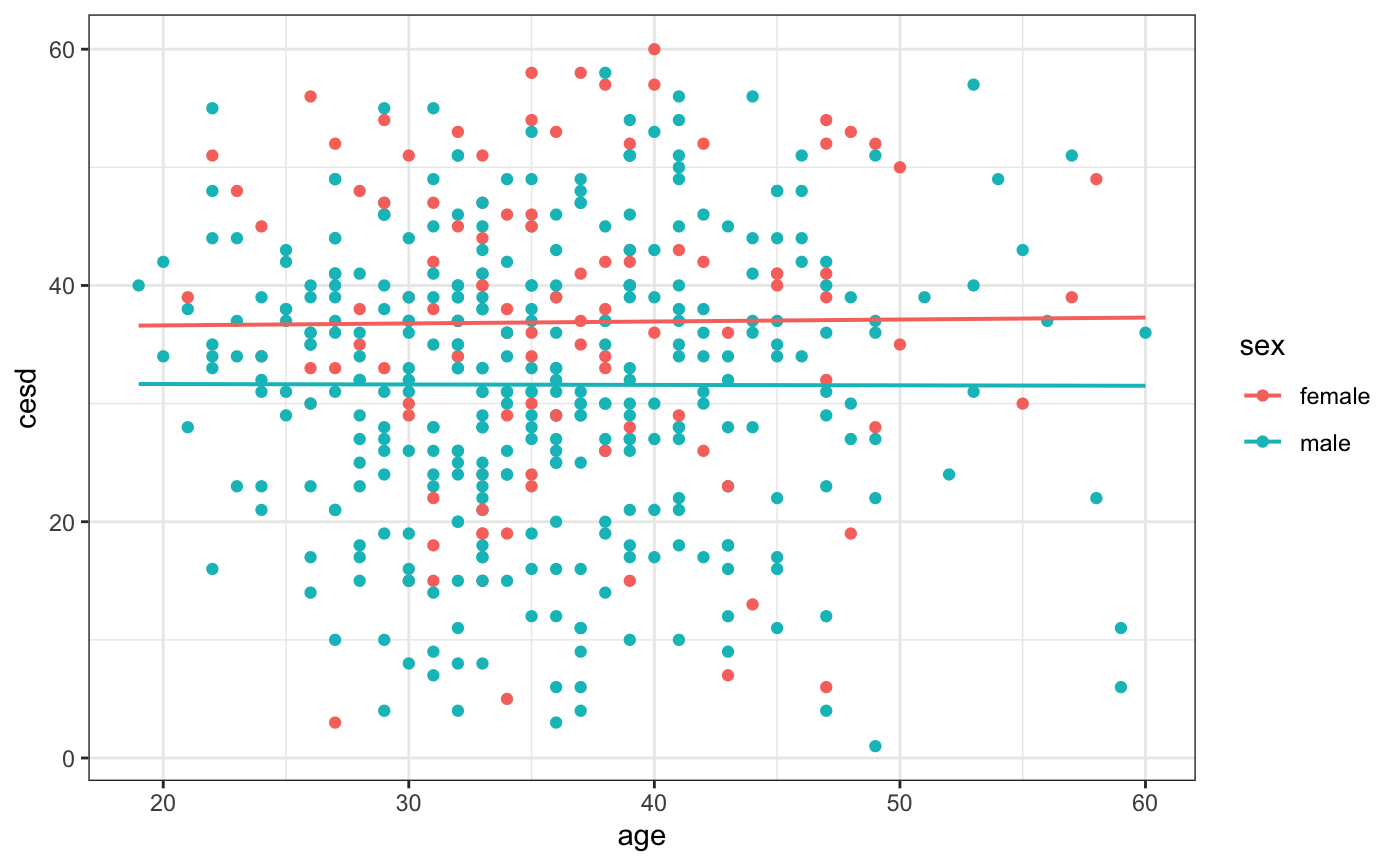### Basic Scatterplot (lattice)

xyplot(cesd ~ age, data = HELPrct)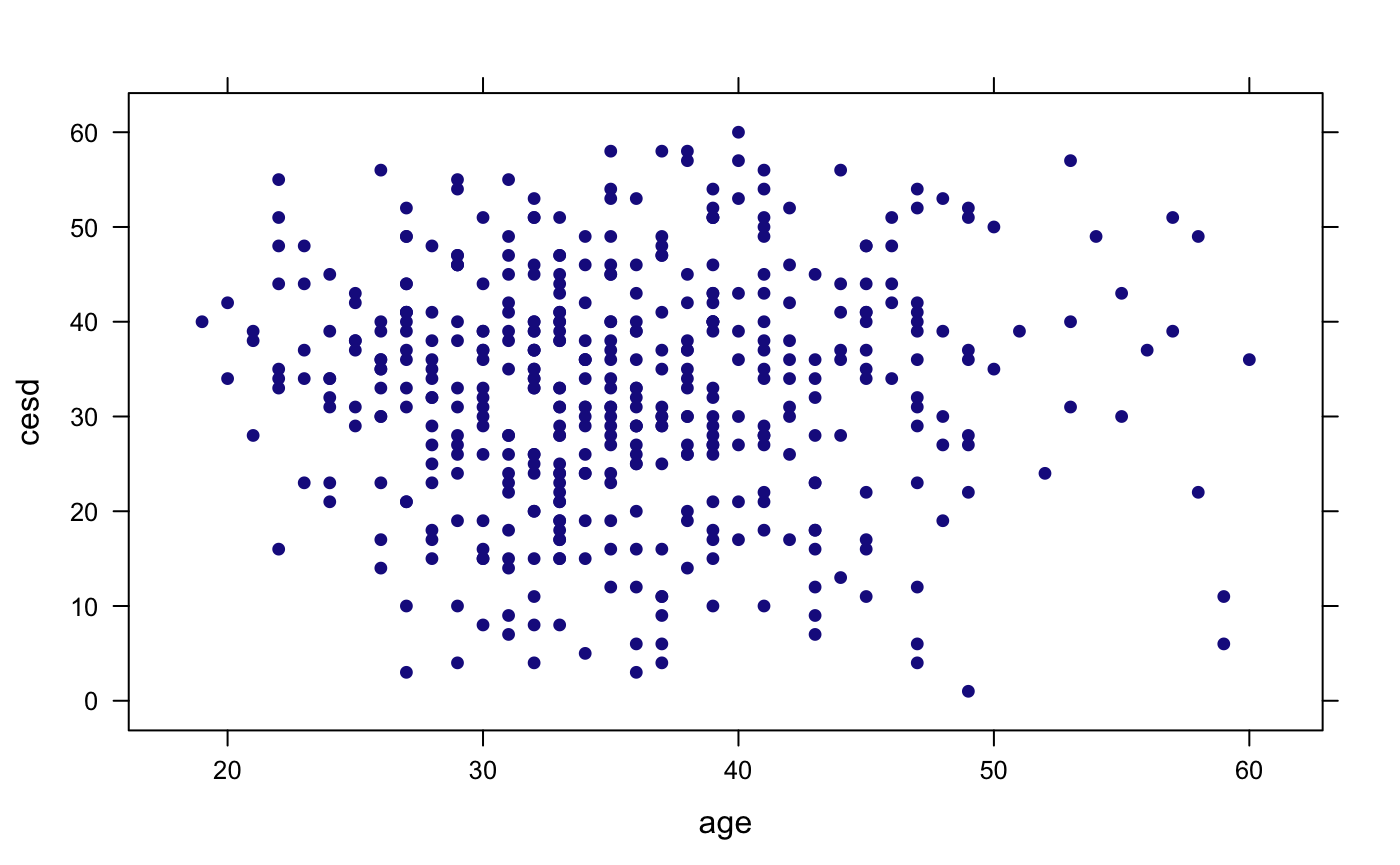### Overlaid scatterplot with linear fit (lattice)

xyplot(cesd ~ age,  data = HELPrct,
groups = sex,
type = c("p", "r"),
auto.key = TRUE)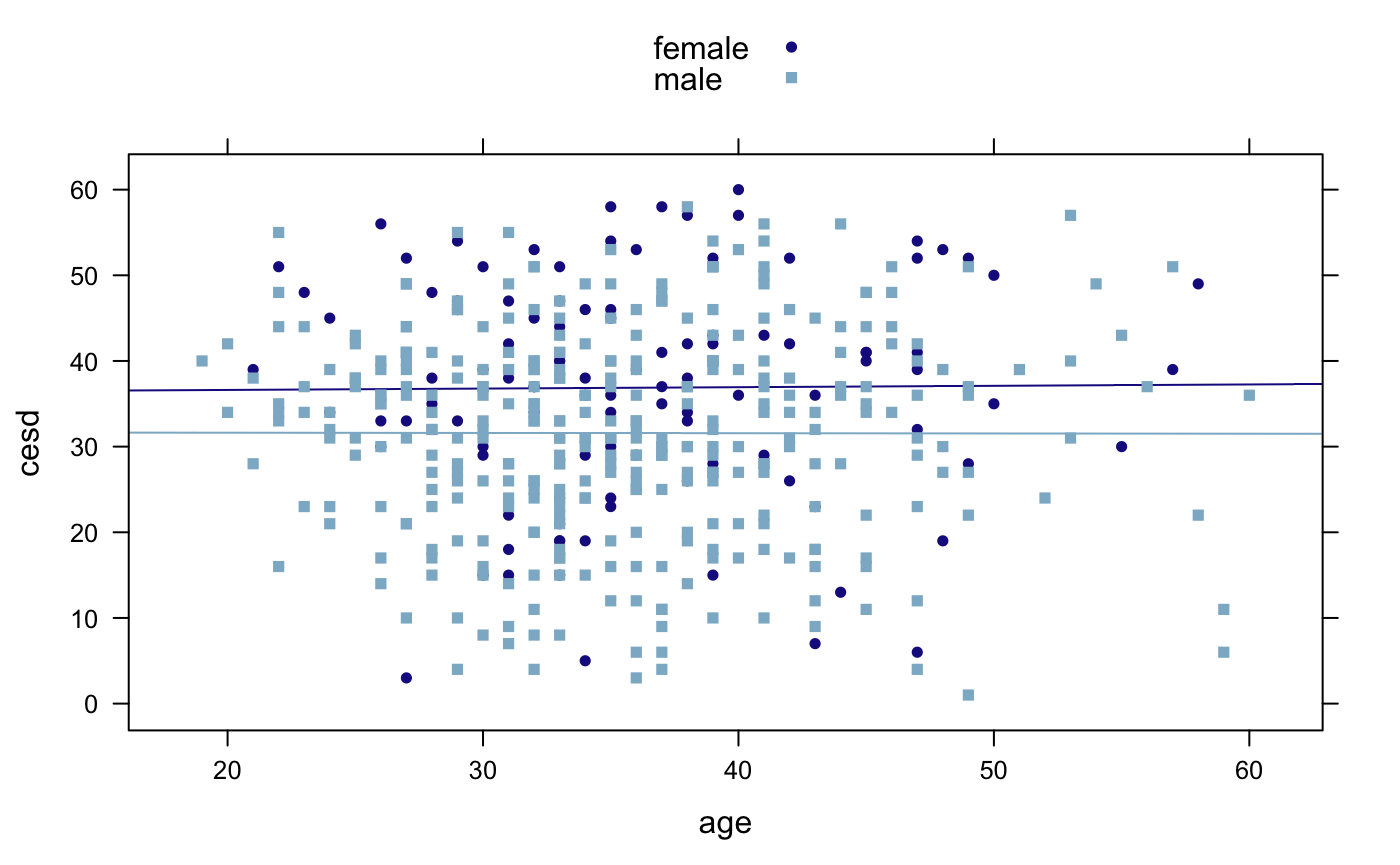### Faceted scatterplot with smooth fit (ggformula)

gf_point(cesd ~ age | sex,
data = HELPrct) %>%
gf_smooth(se = FALSE)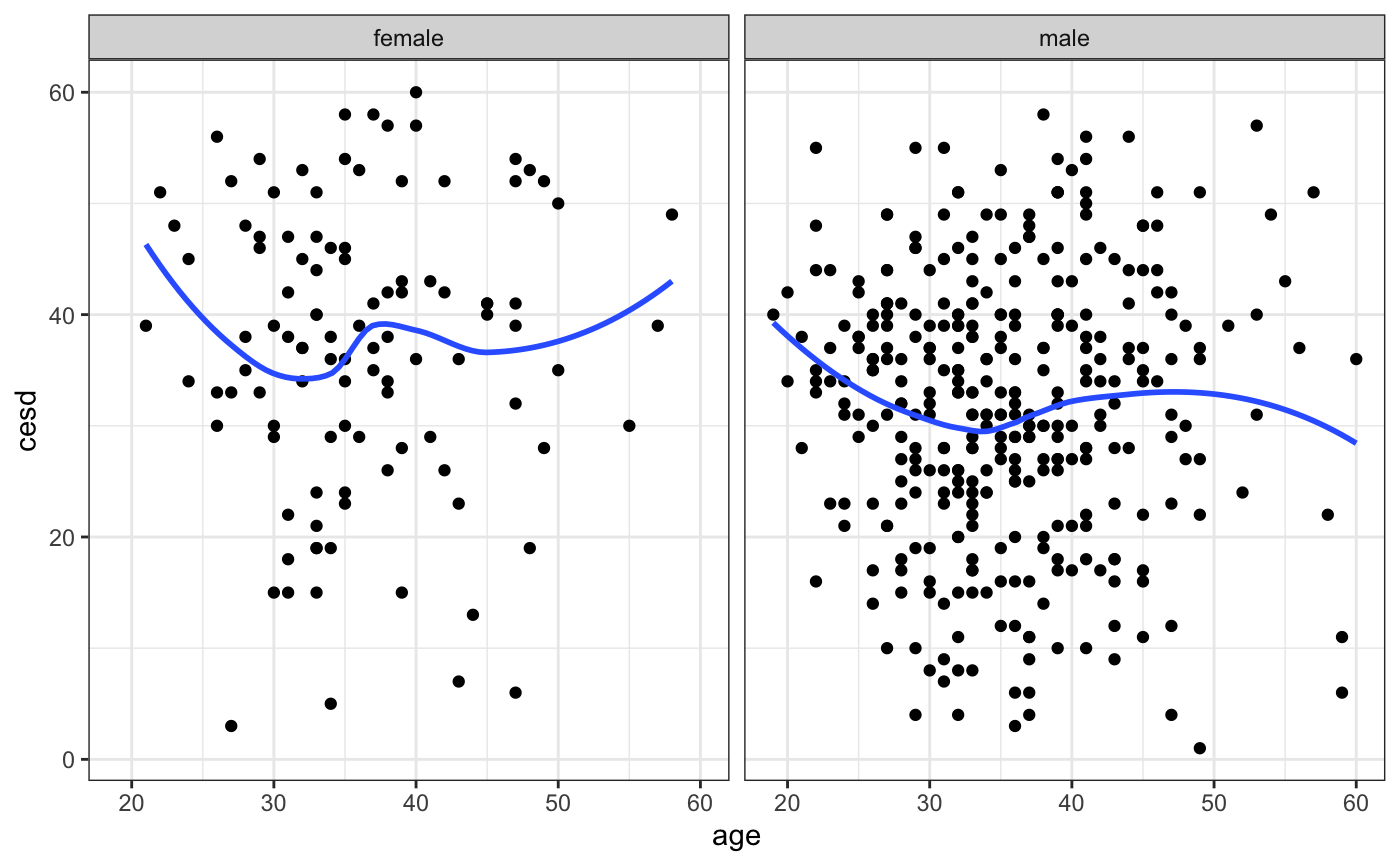## More options for scatterplot with linear fit (ggformula)

gf_point(cesd ~ age, data = HELPrct,
color = ~ sex) %>%
gf_lm() %>%
gf_theme(legend.position = "top") %>%
gf_labs(
title = "This is my ggformula plot",
x     = "age (in years)",
y     = "CES-D measure of
depressive symptoms")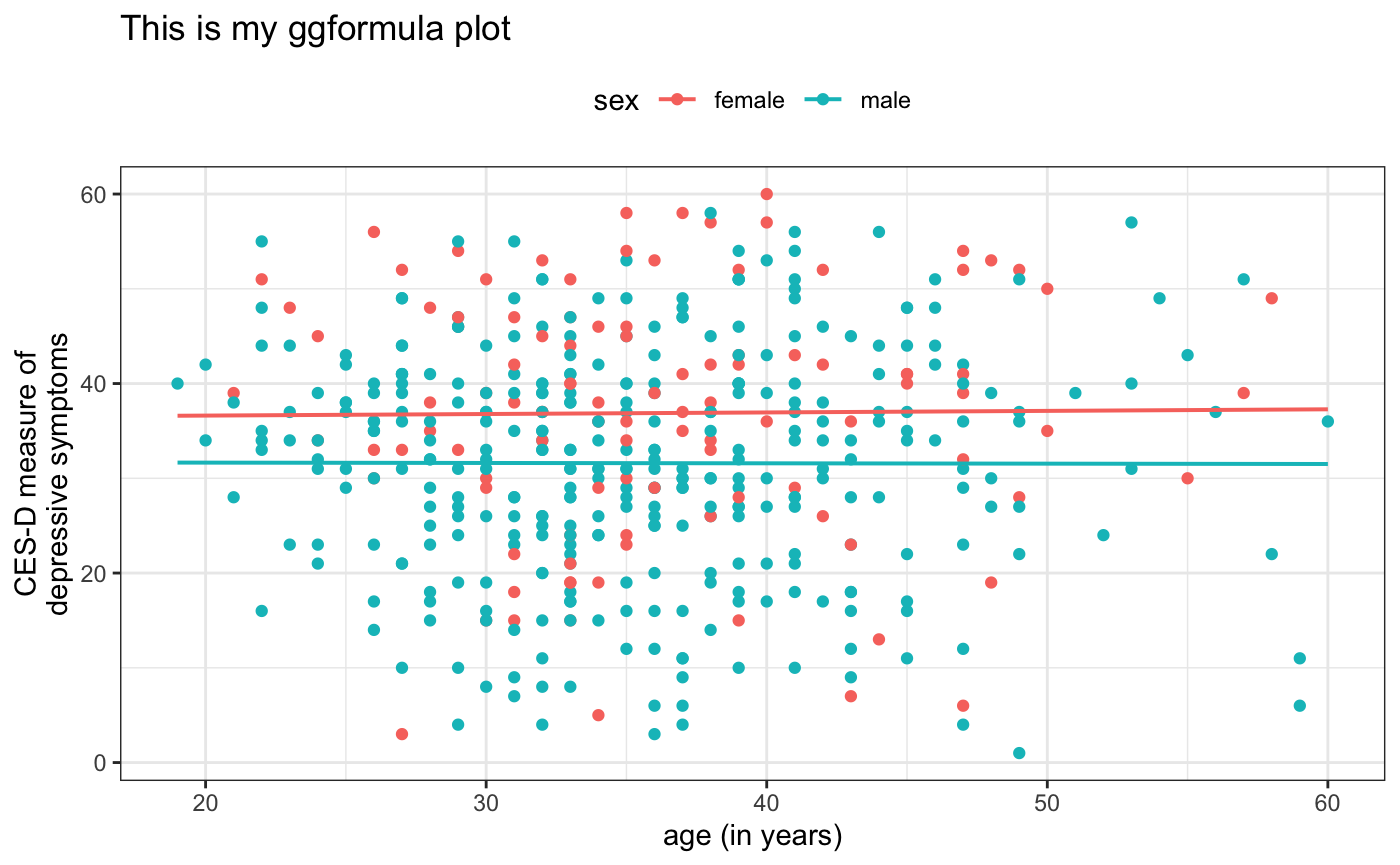## Faceted scatterplot with smooth fit (lattice)

xyplot(cesd ~ age | sex,  data = HELPrct,
type = c("p", "smooth"),
auto.key = TRUE)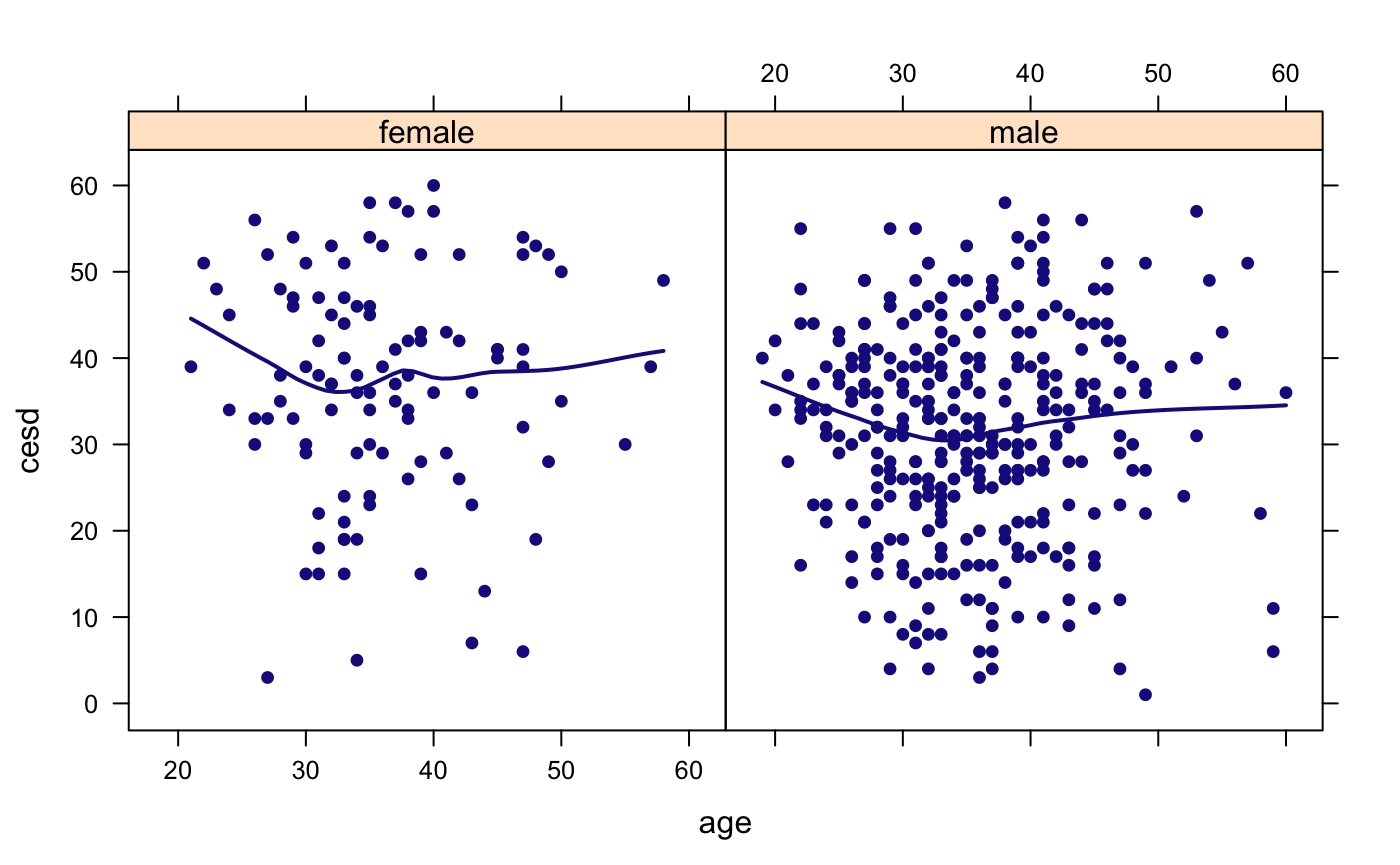## More options for scatterplot with linear fit (lattice)

xyplot(cesd ~ age, groups = sex,
type = c("p", "r"),
auto.key = TRUE,
main = "This is my lattice plot",
xlab = "age (in years)",
ylab = "CES-D measure of
depressive symptoms",
data = HELPrct)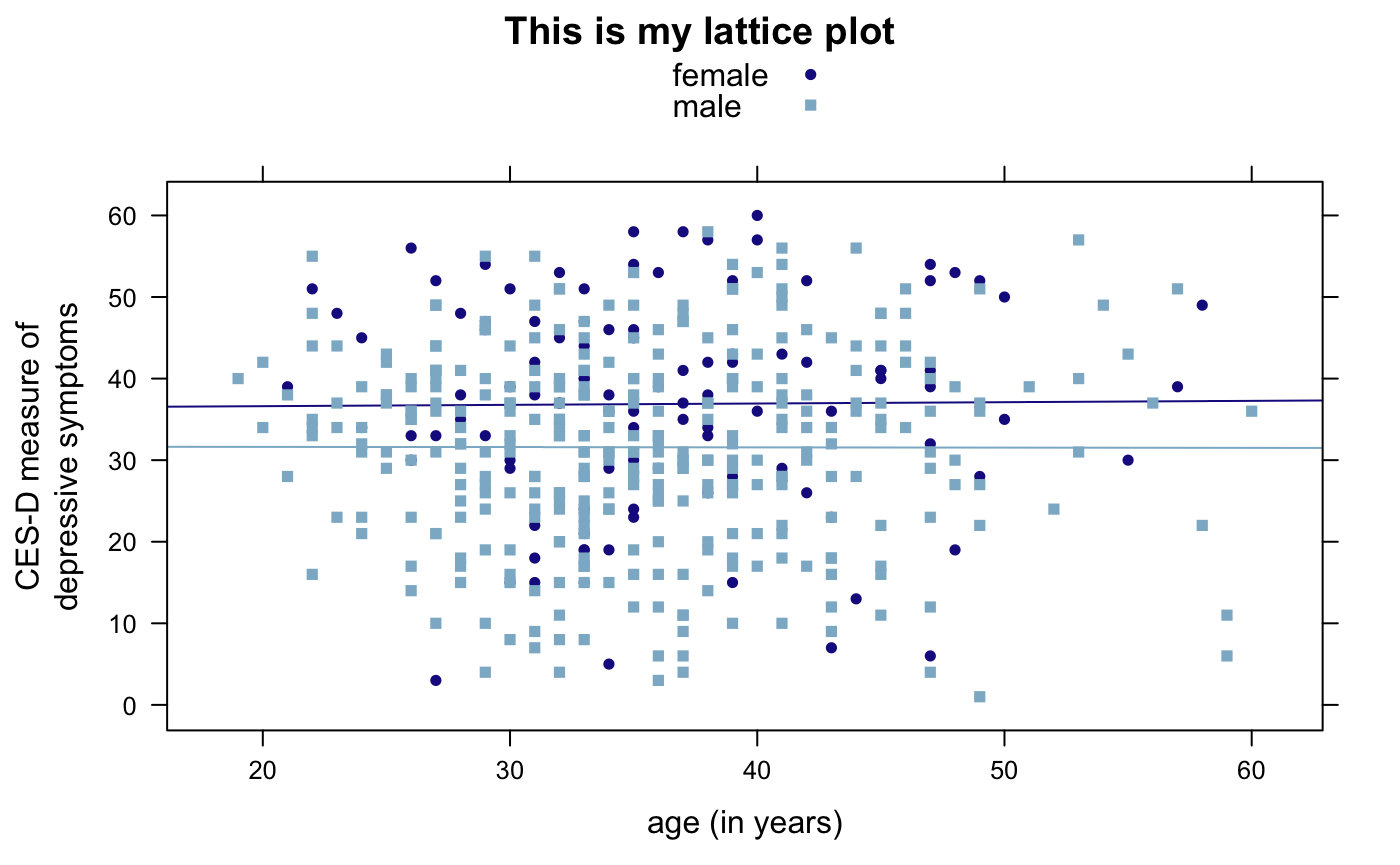## Refining graphs

### Log scales (ggformula)

gf_point(cesd ~ age, data = HELPrct) %>%
gf_refine(scale_y_log10())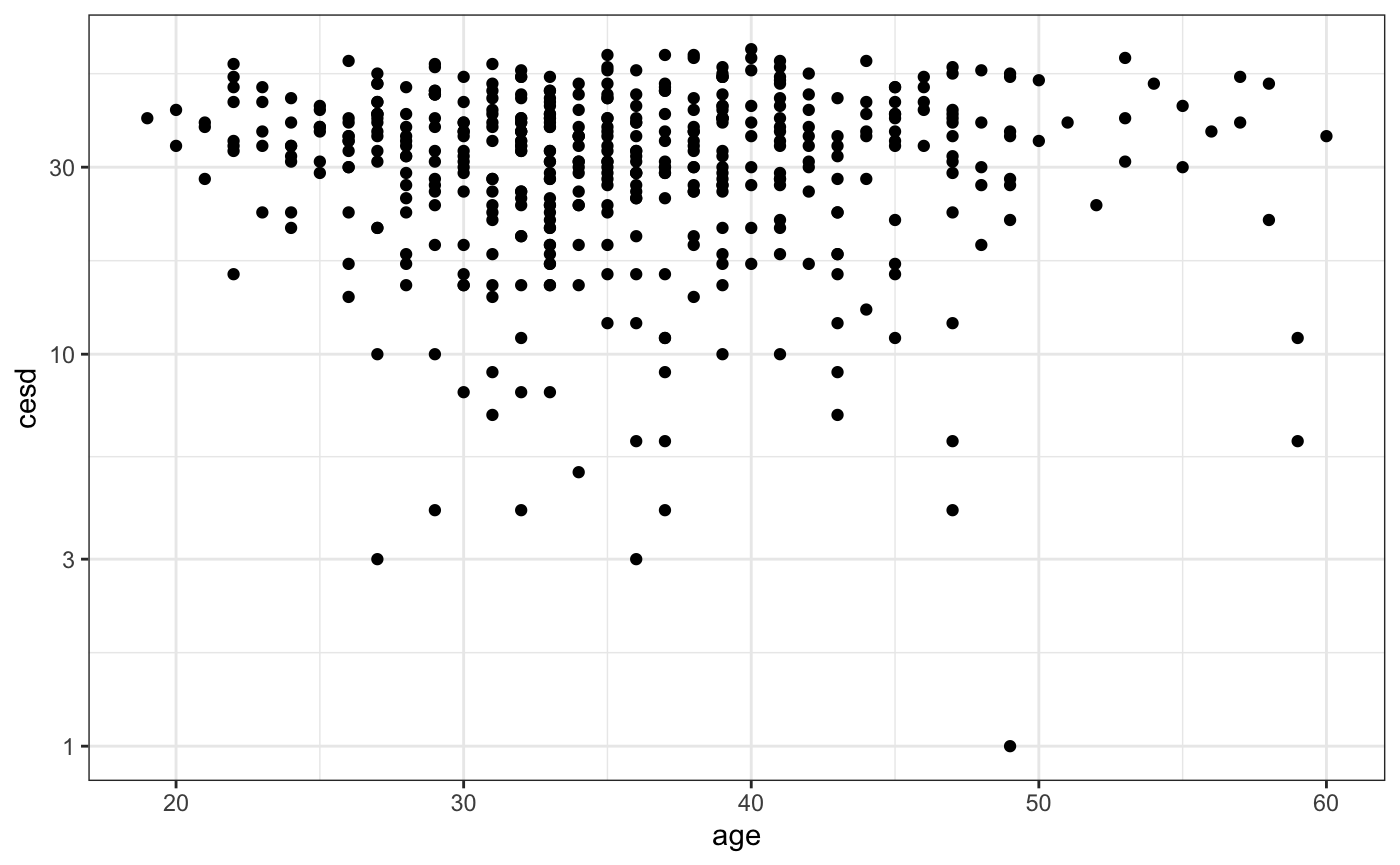### Custom Colors (ggformula)

gf_dens(
~ cesd, data = HELPrct,
color = ~ sex) %>%
gf_rug(
0 ~ cesd,
position = position_jitter(height = 0)
) %>%
gf_refine(
scale_color_manual(
values = c("navy", "red")))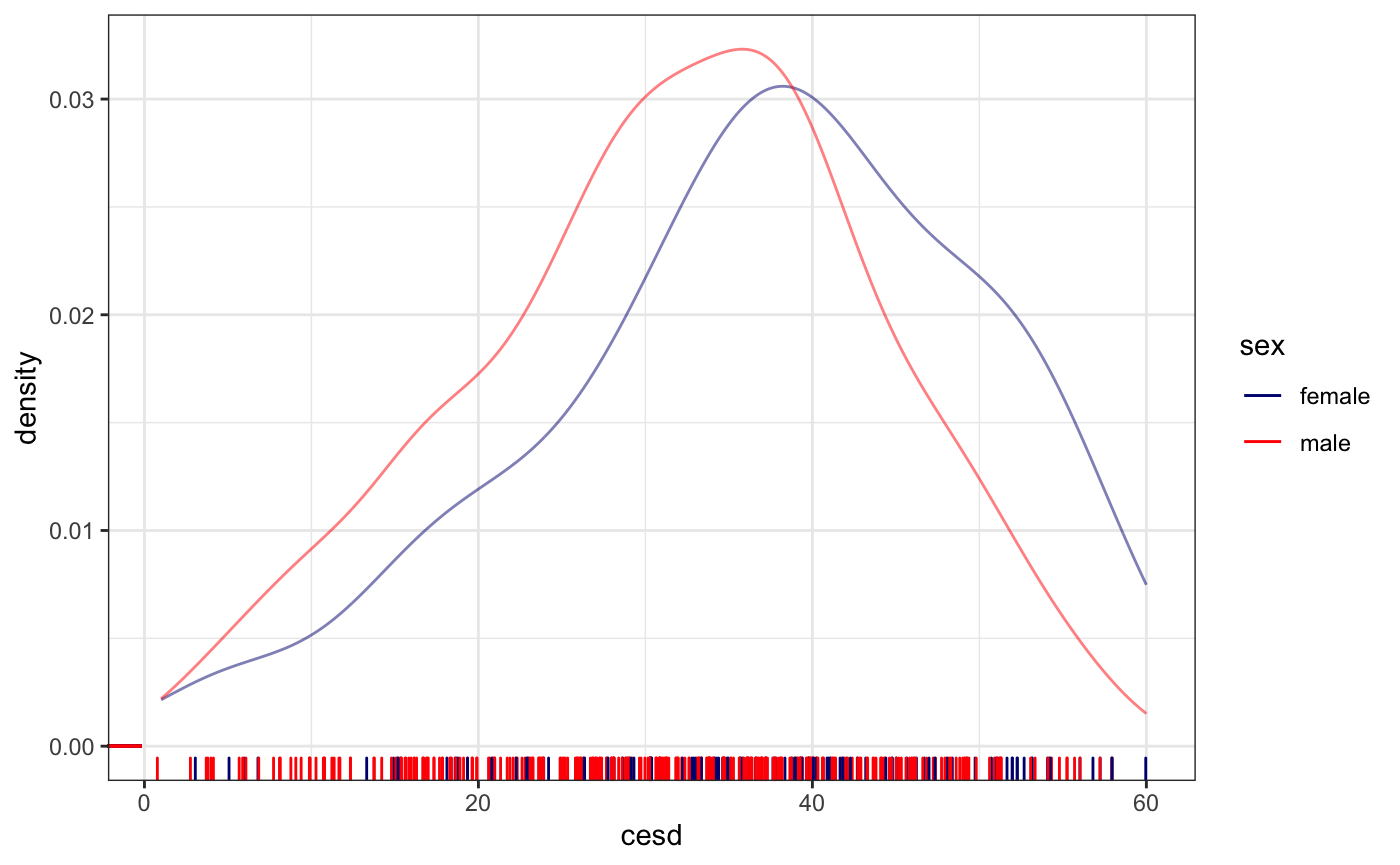### Log scales (lattice)

xyplot(
cesd ~ age, data = HELPrct,
scales = list(y = list(log = TRUE)))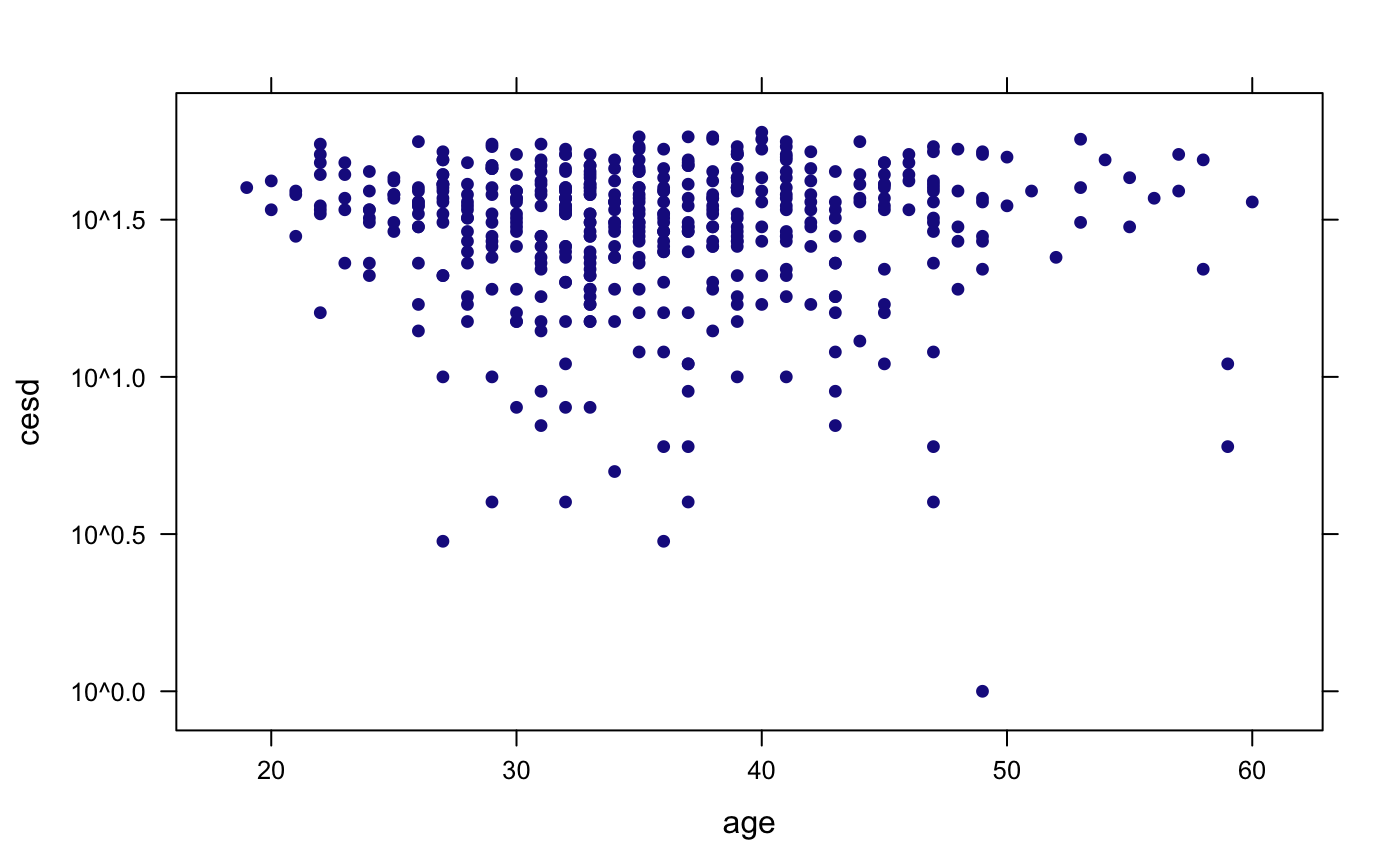### Custom Colors (lattice)

densityplot(
~ cesd, data = HELPrct, groups = sex,
rug = FALSE,
par.settings =
list(
superpose.line =
list(col = c("navy", "red")),
superpose.symbol =
list(col = c("navy", "red"))
))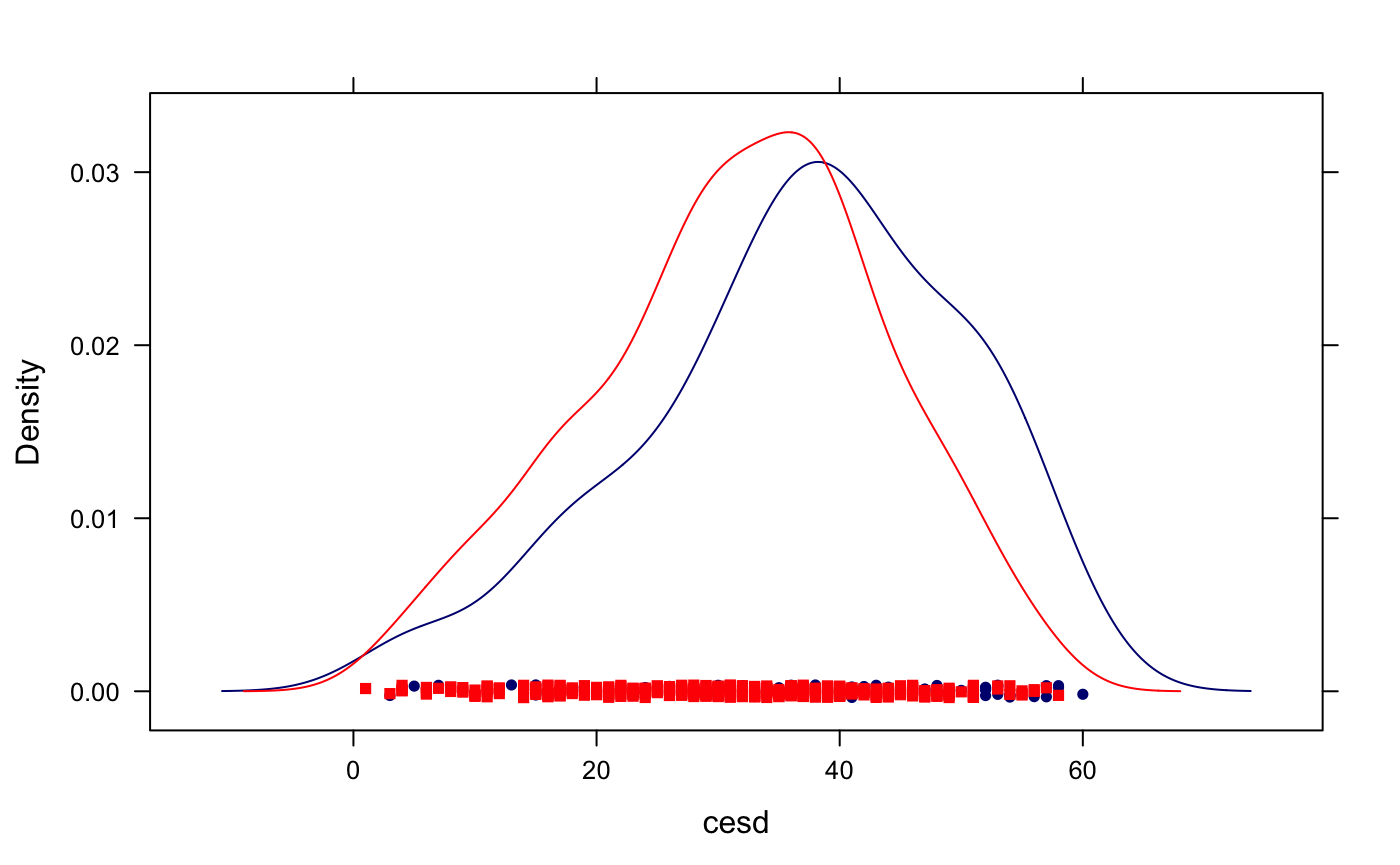## Want to explore more?

Within RStudio, after loading the mosaic package, try running the command mplot(ds) where ds is a dataframe. This will open up an interactive visualizer that will output the code to generate the figure (using lattice, ggplot2, or ggformula) when you click on Show Expression.